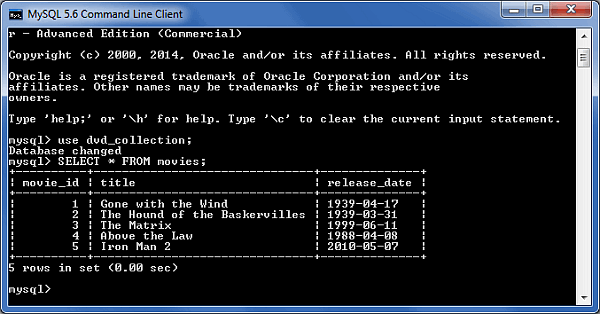# MySQL on CLI

Access monitor: `mysql -u [username] -p;` (will prompt for password)

Show all databases: `show databases;`

Access database: `mysql -u [username] -p [database]` (will prompt for password)

Create new database: `create database [database];`

Select database: `use [database];`

Show all tables: `show tables;`

Show table structure: `describe [table];`

Create new table with columns: `CREATE TABLE [table] ([column] VARCHAR(120), [another-column] DATETIME);`

Adding a column: `ALTER TABLE [table] ADD COLUMN [column] VARCHAR(120);`

Adding a column with an unique, auto-incrementing ID: `ALTER TABLE [table] ADD COLUMN [column] int NOT NULL AUTO_INCREMENT PRIMARY KEY;`

Inserting a record: `INSERT INTO [table] ([column], [column]) VALUES ('[value]', [value]');`

MySQL function for datetime input: `NOW()`

Selecting records: `SELECT * FROM [table];`

Explain records: `EXPLAIN SELECT * FROM [table];`

Selecting parts of records: `SELECT [column], [another-column] FROM [table];`

Counting records: `SELECT COUNT([column]) FROM [table];`

Counting and selecting grouped records: `SELECT *, (SELECT COUNT([column]) FROM [table]) AS count FROM [table] GROUP BY [column];`

Selecting specific records: `SELECT * FROM [table] WHERE [column] = [value];` (Selectors: `<`, `>`, `!=`; combine multiple selectors with `AND`, `OR`)

Select records containing `[value]`: `SELECT * FROM [table] WHERE [column] LIKE '%[value]%';`

Select records starting with `[value]`: `SELECT * FROM [table] WHERE [column] LIKE '[value]%';`

Select records starting with `val` and ending with `ue`: `SELECT * FROM [table] WHERE [column] LIKE '[val_ue]';`

Select a range: `SELECT * FROM [table] WHERE [column] BETWEEN [value1] and [value2];`

Select with custom order and only limit: `SELECT * FROM [table] WHERE [column] ORDER BY [column] ASC LIMIT [value];` (Order: `DESC`, `ASC`)

Updating records: `UPDATE [table] SET [column] = '[updated-value]' WHERE [column] = [value];`

Deleting records: `DELETE FROM [table] WHERE [column] = [value];`

Delete all records from a table (without dropping the table itself): `DELETE FROM [table];` (This also resets the incrementing counter for auto generated columns like an id column.)

Removing table columns: `ALTER TABLE [table] DROP COLUMN [column];`

Deleting tables: `DROP TABLE [table];`

Deleting databases: `DROP DATABASE [database];`

Custom column output names: `SELECT [column] AS [custom-column] FROM [table];`

Export a database dump : `mysqldump -u [username] -p [database] > db_backup.sql`

Use `--lock-tables=false` option for locked tables.

Import a database dump : `mysql -u [username] -p -h localhost [database] < db_backup.sql`

Logout: `exit;`